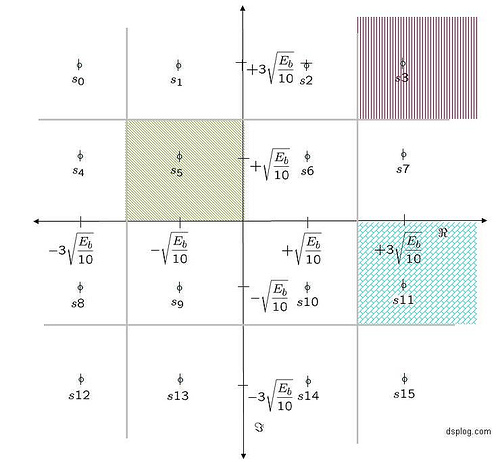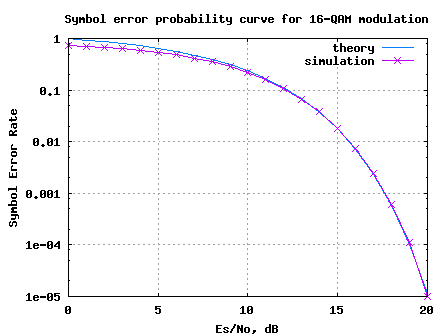- DSP log - http://www.dsplog.com -

Symbol Error Rate (SER) for 16-QAM

Posted By Krishna Sankar On December 9, 2007 @ 12:25 pm In Modulation | 61 Comments

Given that we have went over the symbol error probability for 4-PAM  and symbol error rate for 4-QAM  , let us extend the understanding to find the symbol error probability for 16-QAM (16 Quadrature Amplitude Modulation). Consider a typical 16-QAM modulation scheme where the alphabets (Refer example 5-37 in [DIG-COMM-BARRY-LEE-MESSERSCHMITT]).

are used.

The average energy of the 16-QAM constellation is (here ). The 16-QAM constellation is as shown in the figure belowFigure: 16-QAM constellation

## Noise model

Assuming that the additive noise follows the Gaussian probability distribution function,

with and .

## Computing the probability of error

Consider the symbol in the inside, for example

The conditional probability distribution function (PDF) of given was transmitted is:

.

As can be seen from the above figure, the symbol is decoded correctly only if falls in the area in the black hashed region i.e.

.

Using the equations from (symbol error probability of 4-PAM  as reference)

.

The probability of being decoded incorrectly is,

.

Consider the symbol in the corner, for example

The conditional probability distribution function (PDF) of given was transmitted is:

.

As can be seen from the above figure, the symbol is decoded correctly only if falls in the area in the red hashed region i.e.

.

Using the equations from (symbol error probability of 4-QAM  as reference)

.

The probability of being decoded incorrectly is,

.

Consider the symbol which is not in the corner OR not in the inside, for example

The conditional probability distribution function (PDF) of given was transmitted is:

.

As can be seen from the above figure, the symbol is decoded correctly only if falls in the area in the blue hashed region i.e.

.

Using the above two cases are reference,

.

The probability of being decoded incorrectly is,

.

Total probability of symbol error

Assuming that all the symbols are equally likely (4 in the middle, 4 in the corner and the rest 8), the total probability of symbol error is,

.

## Simulation model

Simple Matlab/Octave code for generating 16QAM constellation, transmission through AWGN channel and computing the simulated symbol error rate.

Click here to download : Matlab/Octave script for simulating 16QAM symbol error rateFigure: Symbol Error Rate curve for 16QAM modulation

Observations

1. Can observe that for low values, the theoretical results seem to be ‘pessimistic’ ‘optimistic’ compared to the simulated results. This is because for the approximated theoretical equation, the term was ignored. However, this approximation is valid only when the term is small, which need not be necessarily true for low values.

## Reference

[DIG-COMM-BARRY-LEE-MESSERSCHMITT]Digital Communication: Third Edition, by John R. Barry, Edward A. Lee, David G. MesserschmittHope this helps.

Krishna

URL to article: http://www.dsplog.com/2007/12/09/symbol-error-rate-for-16-qam/

URLs in this post:

 symbol error probability for 4-PAM: http://www.dsplog.com/2007/10/07/symbol-error-rate-for-pam/

 symbol error rate for 4-QAM: http://www.dsplog.com/2007/11/06/symbol-error-rate-for-4-qam/